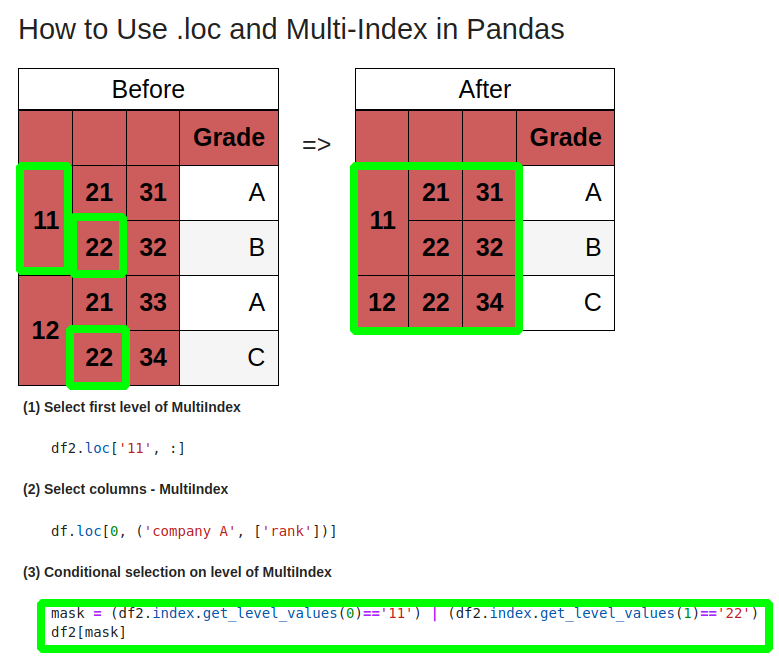In this tutorial, we'll see how to select values with `.loc()` on multi-index in Pandas DataFrame.

Here are quick solutions for selection on multi-index:

(1) Select first level of MultiIndex

``````df2.loc['11', :]
``````

(2) Select columns - MultiIndex

``````df.loc[0, ('company A', ['rank'])]
``````

(3) Conditional selection on level of MultiIndex

``````mask = (df2.index.get_level_values(0)=='11') | (df2.index.get_level_values(1)=='22')
``````

## Setup

• first one with multi index on columns
• second one with multi index on rows
``````import pandas as pd

cols = pd.MultiIndex.from_tuples([('company A', 'rank'), ('company A', 'points'), ('company B', 'rank'), ('company B', 'points')])
df = pd.DataFrame([[1,2,3,4], [2,3, 3,4]], columns=cols)
``````

data:

company A company B
rank points rank points
0 1 2 3 4
1 2 3 3 4

The second one:

``````df2 = pd.DataFrame(
index=[
["11", "11", "12", "12"],
["21", "22", "21", "22"],
["31", "32", "33", "34"]
]
)
``````

data

11 21 31 A
22 32 B
12 21 33 A
22 34 C

## Step 1: .loc() and MultiIndex

Pandas method `.loc()` can select on multi-index.

To find out what are the index values we can use method: `df2.index` which will give us:

``````MultiIndex([('11', '21', '31'),
('11', '22', '32'),
('12', '21', '33'),
('12', '22', '34')],
)
``````

To select values from the multi index above we can use following syntax:

``````df2.loc[('11', '21', '31'), :]
``````

which give us:

``````Grade    A
Name: (11, 21, 31), dtype: object
``````

## Step 2: Select first level of multi-index

To select first level of multiindex we can use method `loc()` and provide list of values:

``````df2.loc[['11']]
``````

result:

11 21 31 A
22 32 B

To select multiple values from the first level we can use:

``````df2.loc[['11', '12']]
``````

## Step 3: Select second level of multi-index

To select second or N-th level from multi index in Pandas DataFrame we can use `slice(None)` or method `get_level_values()`:

``````df2[df2.index.get_level_values(1)=='21']
``````

or

``````sel = (slice(None), ['21'], slice(None))
df2.loc[sel]
``````

result in selection of the second level of this multi-index:

11 21 31 A

Alternatively we can create IndexSlice object:

``````idx = pd.IndexSlice
df2.loc[idx[:,['21'],:],:]
``````

to get the same result.

## Step 4: Conditional selection on multi-index

For conditional selection on multi index in pandas we can use method `get_level_values()` and mask:

``````mask = (df2.index.get_level_values(0)=='11') | (df2.index.get_level_values(1)=='22')
``````

In this way we can combine multiple conditions:

• select first level - value '11'
• or second level - value '22'
11 21 31 A
22 32 B
12 22 34 C## Step 5: Query selection on multi-index

If our multi-index has named levels we can use queries to select data:

``````df.query('level1 == "11" | level2 == "21"')
``````

## Step 6: Select multi-index column

All from above apply to columns. For columns we need to pass criteria as second value:

``````df.loc[0, ('company A', ['rank'])]
``````

result:

``````company A  rank    1
Name: 0, dtype: int64
``````

## Conclusion

In this article, we looked at different solutions for selection and quering data from Pandas Multi-Index. We focused on row/index selection, column selection is exactly the same.

We covered conditional selection, selection from first or second level and queries on Multi-Index DataFrame.